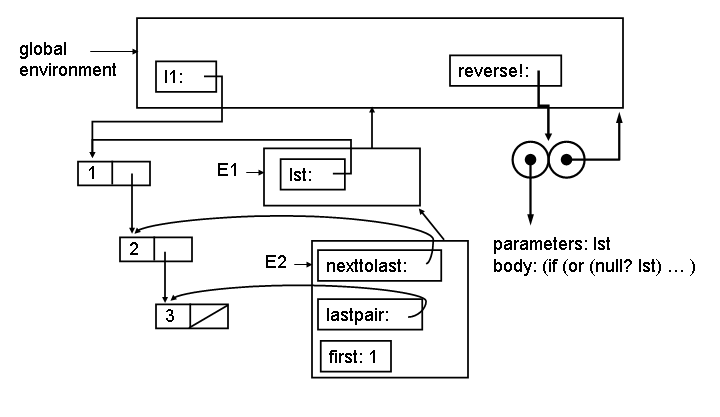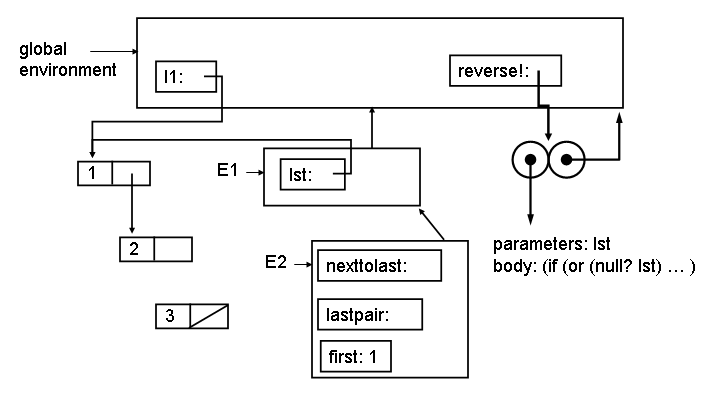University of Virginia, Department of Computer Science CS200: Computer Science, Spring 2004

## Exam 2 Study Guide

Exam 2 will be handed out on Wednesday, 14 April and due on Monday, 19 April.

It will likely contain questions on:

• Programming with mutation, environment model of evaluation
• Object-oriented programming
• Measuring work using orders of growth
• Computability
• Databases
• Networks
• Finite state machines
• Turing machines
• Lambda Calculus
• Modeling computation
This document contains selected questions from previous Exam 2 and Final Exams that are covered by the upcoming Exam 2. You can find the answers to these questions on the comments from previous exams posted at http://www.cs.virginia.edu/cs200/exams/.

### Mutation and Objects

(From Spring 2003 Exam 2)

Consider the classes defined below: sail-boat is a subclass of boat which is a subclass of object.

```(define (ask object message . args)
(apply (get-method object message) object args))

(define (get-method object message)
(object message))

(define make-object
(lambda (name)
(lambda (message)
(case message
((class) (lambda (self) 'object))
((object?) (lambda (self) #t))
((name) (lambda (self) name))
(else (error "No method"))))))

(define make-boat
(lambda (name)
(let ((super (make-object name))
(floating #t))
(lambda (message)
(case message
((class) (lambda (self) 'boat))
((boat?) (lambda (self) #t))
((is-floating?) (lambda (self) floating))
((sink) (lambda (self) (set! floating #f)))
(else (get-method super message)))))))

(define make-sail-boat
(lambda (name)
(let ((super (make-boat name))
(sailing #f))
(lambda (message)
(case message
((class) (lambda (self) 'sail-boat))
((raise-sail) (lambda (self) (set! sailing #t)))
((lower-sail) (lambda (self) (set! sailing #f)))
(else (get-method super message)))))))
```
1. The following environment diagram shows the state after evaluating (define discovery (make-sail-boat "discovery")). The procedure associated with the name discovery is missing its environment pointer. Add the missing environment pointer to the diagram.

2. There are three frames in the diagram (labeled F1, F2 and F3) that contain a place named name with the value "discovery". If we evaluate (ask discovery 'name) in the global environment, in which frame with the value of name be found?

### Objects and Environments

(From Spring 2002 Final)

Cy D. Fect defines a procedure reverse! that uses mutation to reverse a list:

```(define (next-to-last-pair lst)
(if (null? lst) (error "Null list!")
(if (null? (cdr lst)) (error "Single length list!")
(if (null? (cdr (cdr lst))) lst
(next-to-last-pair (cdr lst))))))

(define (reverse! lst)
(if (or (null? lst) (eq? 1 (length lst)))
#f ;; Nothing to do for empty or length 1 list (value doesn't matter)
(let* ((nexttolast (next-to-last-pair lst))
(lastpair (cdr nexttolast))
(first (car lst)))
;;; Point 0
(set-cdr! nexttolast null)
;;; Point 1
(reverse! (cdr lst))
(set-cdr! nexttolast lastpair)
(set-car! lst (car lastpair))
;;; Point 2
(set-car! lastpair first))))
```
Consider evaluating:

> (define ilist (list 1 2 3))

> (reverse! ilist)

> ilist

(3 2 1)

At Point 0 on the first call to reverse! the environment looks like:The E1 environment is created by the application (reverse! ilist). The E2 environment is created by the let inside reverse! (which desugars to an application of a lambda). (The let* actually desugars into more than one lambda, and more than one environment, but for this question we combine them into one environment.)

4. Show what the environment looks like at Point 1 (after evaluating (set-cdr! nexttolast null)). To make your drawing easier, here is a template drawing. You can answer this question by only adding to this template.5. Show what the environment looks like at Point 2. You can answer this question by only adding to this template.### Computability

(Based on Spring 2003 Exam 2, Question 6)

Is the Greater than Zero Problem described below decidable?

Input: A program P and input n
Output: If evaluating P applied to n would produce a value greater than 0, true. If evaluating P applied to n would produce a value that is not a number, or is a number less than or equal to 0, or would not terminate, output is false.

### Lambda Calculus

(From Spring 2003 Exam 2)

In class, we defined if, T and F using Lambda Calculus as:

if ≡ λ pca . p c a
T ≡ λ xy . x
F ≡ λ xy . y
Suppose we instead defined if as:
if ≡ λ pca . p a c

8. Show how T and F would need to be defined to make the new definition of if work correctly.

### Lambda Calculus

(From Spring 2002 Final)

7. Given T, F and if as defined in class:

T ≡ λ xy . x)
F ≡ λ xy . y)
if ≡ λ pca . pca
a. Define a Lambda Calculus term that behaves like not. For example, if (not T) M N should reduce to N and if (not F) M N should reduce to M.

b. Show that your definition of not works by showing the steps to reduce if (not T) M N to normal form (where M and N represent and Lambda Calculus term in normal form).

### Universal Language

(From Spring 2003 Exam 2)

Consider the preSQL language described below:

Commands ::= Command ; Commands
Commands ::= Command
Command ::= CreateTableCommand | InsertCommand | CountCommand | DeleteCommand

CreateTable ::= CREATE TABLE Name
Create a new table named Name with no entries.
InsertCommand ::= INSERT INTO Table Value
Insert a value (TRUE or FALSE) as the last element in table Table.
Value ::= TRUE
Value ::= FALSE
CountCommand ::= COUNT Table
Evaluates to the number of entries in Table.
DeleteCommand ::= DELETE FROM Table
Remove the first (earliest inserted) element from the table Table.

PreSQL is similar to SQL except:
• Entries in a table have one value (TRUE or FALSE). There are no fields, since each table entry is only one value. (Note that there is no way to get the actual values of entries.)
• The DELETE command just deletes the first entry in the table.
• There is no SELECT command and no UPDATE command.
• The COUNT command can be used to obtain the number of entries in a table.
Here is an example sequence of interactions in PreSQL:

> CREATE TABLE tab

> INSERT INTO tab TRUE

> INSERT INTO tab FALSE

> COUNT tab

2

> DELETE FROM tab

> COUNT tab

1

9. Is PreSQL a Universal Language? Use convincing arguments to support your answer.

Command ::= UNTILEMPTY Table { Commands }
UNTILEMPTY will check if Table is empty (that is, it has zero entries). If it is empty, it does nothing. Otherwise, it will execute the Commands and repeat the UNTILEMPTY command. It will keep repeating the Commands until the Table is empty.

For example, UNTILEMPTY tab { DELETE FROM tab } would delete all entries from that table tab.

10. Show how you could do addition using PreSQLsql. Your addition code should assume the input is in the form of two tables, tableA and tableB. After addition, a new table named output should have the result. Provide code that could be used for the missing code below that would correctly add any two natural numbers (your code may modify the input tables):

 > COUNT tableA n > COUNT tableB m > insert your code here > COUNT output Value of n + m

### Models of Computation

(From Spring 2002 Final)

Phine Knight suggests the modelling computation using a Phine Machine consisting of a list of numbered instructions (the Instructions), a pointer to the current instructions (the InstructionPointer), and a store that associates name and values (the Store).

A program executes by executing the instruction numbered by the InstructionPointer, and then increasing the InstructionPointer by 1. This continues unless the Instruction is HALT.

Instructions can do one of four things:

• Set the value associated with a name to zero (e.g., X := 0)
• Set the value associated with a name to the value of a name plus 1 (e.g., Y := X - 1)
• Set the value associated with a name to the value of a name minus 1 (e.g., X := X - 1)
• Halt (e.g., HALT)
We can describe the state of a Phine Machine by listing the Instructions, InstructionPointer and Store. For example, here is a Phine Machine with four instructions and an empty store:
```   ( <1: X := 0
2: X := X + 1
3: Y := X - 1
4: HALT >,
1,
{ } )
```
The model will start by executing instruction number 1, which puts <X, 0> in the store and advances the Instruction Pointer to 2. After this, the state is:
```   ( <1: X := 0
2: X := X + 1
3: Y := X - 1
4: HALT >,
2,
{ <X, 0> } )
```
After the next two steps, the state will be:
```   ( <1: X := 0
2: X := X + 1
3: Y := X - 1
4: HALT>,
4,
{ <X, 1>, <Y, 0> } )
```
8. Write a BNF grammar that could be used to describe the state of a Phine Machine. A good answer will describe the smaller language possible that includes strings for describing all possible Phine Machine states.

9. Is this Phine Machine model of computation equivalent to a Turing Machine? Argue convincingly why it is or isn't.

10. Condy Shonal suggests adding one new instruction to Knight's model:

```    Instruction ::= IF Name = 0 GOTO Number
```
If the value of Name in the Store is 0, then this instruction sets the InstructionPointer to Number. If it is not, then this instruction does nothing and advances the InstructionPointer by one.

For example,

```    ( <1: IF A = 0 GOTO 5
2: B := B + 1
3: A := A - 1
4: GOTO 1
5: HALT>,
1,
{ <A, n>, <B, m> } )
```
is a program that will halt with B having the value n + m.

Condy claims her new model of computation is as powerful as a Turing Machine, but Fine Knight does not believe her. Write an informal but convincing argument that this model is as powerful as a Turing Machine.